# [Solved] Write the Nuclear Equation for the Alpha Decay of

Question 9
Multiple Choice

## Write the nuclear equation for the alpha decay of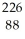Ra.

A)Ra +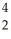He →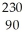Th
B)Ra →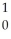n +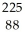Ra
C)Ra →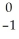e +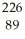Ac
D)Ra +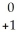e →Ac
E)Ra →He +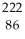Rn

10+ million students use Quizplus to study and prepare for their homework, quizzes and exams through 20m+ questions in 300k quizzes.

### Chemistry

Explore our library and get Introductory / Preparatory Chemistry Homework Help with various study sets and a huge amount of quizzes and questions

145

Study sets

3.2K

Quizzes

275.8K

Questions

Upload material to get free accessInvite a friend and get free accessSubscribe and get an instant access